# Trigonometry

If you know that cos(γ) = sin (806°), what is the angle γ?

γ =  4 °

### Step-by-step explanation:

$\gamma =\frac{180}{\pi }\mathrm{arccos}\left(\mathrm{sin}\left(80{6}^{\circ }\right)\right)=4\text{°}$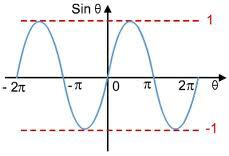Did you find an error or inaccuracy? Feel free to write us. Thank you!Tips to related online calculators
Most natural application of trigonometry and trigonometric functions is a calculation of the triangles. Common and less common calculations of different types of triangles offers our triangle calculator. Word trigonometry comes from Greek and literally means triangle calculation.

## Related math problems and questions:

• Trigonometric functionsIn the right triangle is: tg α= frac(2) 1 Find the value of s and c: sin α= (s)/(√ 5) cos α= (c)/(√ 5)
• CotangentIf the angle α is acute, and cotan α = 1/3. Determine the value of sin α, cos α, tg α.
• Q-Exam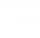If tg α = 9.6, Calculating sin α, cos α, cotg α .
• Substitution method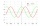Solve goniometric equation: sin4 θ - 1/cos2 θ=cos2 θ - 2
• CosineThe point (3, 4) is on the terminal side of angle θ. cos θ = ...
• Sin cos tan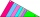In triangle ABC, right-angled at B. Sides/AB/=7cm, /BC/=5cm, /AC/=8.6cm. Find to two decimal places. A. Sine C B. Cosine C C. Tangent C.
• What percentageWhat percentage of the Earth’s surface is seen by an astronaut from a height of h = 350 km. Take the Earth as a sphere with the radius R = 6370 km
• TrigonometryIs true equality? sin(x +13 π)= sin(x)
• SinesIn ▵ ABC, if sin(α)=0.5 and sin(β)=0.6 calculate sin(γ)
• Greatest angleCalculate the greatest triangle angle with sides 197, 208, 299.
• Cablecar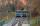Funicular on Petrin (Prague) was 408 meters long and overcomes the difference 106 meters in altitude. Calculate the angle of climb.
• Right angled triangle 3Side b = 1.5, hypotenuse angle A = 70 degrees, Angle B = 20 degrees. Find its unknown sides length.
• Roof angle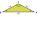The roof of the house has the shape of an isosceles triangle with arms 4 m long and the size of the base 6 m. How big an angle alpha does its roof make?
• SSA and geometryThe distance between the points P and Q was 356 m measured in the terrain. The PQ line can be seen from the viewer at a viewing angle of 107° 22 '. The observer's distance from P is 271 m. Determine the viewing angle of P and observer.
• Isosceles triangle 8If the rate of the sides an isosceles triangle is 7:6:7, find the base angle correct to the nearest degree.
• Ratio iso triangleThe ratio of the sides of an isosceles triangle is 7:6:7 Find the base angle to the nearest answer correct to 3 significant figure.
• Trapezoid MOThe rectangular trapezoid ABCD with the right angle at point B, |AC| = 12, |CD| = 8, diagonals are perpendicular to each other. Calculate the perimeter and area of ​​the trapezoid.